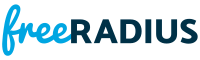`#include <freeradius-devel/libradius.h>`
`#include <freeradius-devel/md5.h>`Include dependency graph for radius_decode.c:

Go to the source code of this file.

## Functions

static ssize_t decode_concat (TALLOC_CTX *ctx, vp_cursor_t *cursor, fr_dict_attr_t const *parent, uint8_t const *data, size_t const packet_len)
Convert a "concatenated" attribute to one long VP. More...

static ssize_t decode_extended (TALLOC_CTX *ctx, vp_cursor_t *cursor, fr_dict_attr_t const *parent, uint8_t const *data, size_t attr_len, size_t packet_len, void *decoder_ctx)
Convert a fragmented extended attr to a VP. More...

static ssize_t decode_vsa (TALLOC_CTX *ctx, vp_cursor_t *cursor, fr_dict_attr_t const *parent, uint8_t const *data, size_t attr_len, size_t packet_len, void *decoder_ctx)
Convert a top-level VSA to one or more VPs. More...

static ssize_t decode_vsa_internal (TALLOC_CTX *ctx, vp_cursor_t *cursor, fr_dict_attr_t const *parent, uint8_t const *data, size_t data_len, void *decoder_ctx, fr_dict_vendor_t const *dv)
Convert a top-level VSA to a VP. More...

static ssize_t decode_wimax (TALLOC_CTX *ctx, vp_cursor_t *cursor, fr_dict_attr_t const *parent, uint8_t const *data, size_t attr_len, size_t packet_len, void *decoder_ctx, uint32_t vendor)
Convert a Vendor-Specific WIMAX to vps. More...

ssize_t fr_radius_decode_pair (TALLOC_CTX *ctx, vp_cursor_t *cursor, fr_dict_attr_t const *parent, uint8_t const *data, size_t data_len, void *decoder_ctx)
Create a "normal" VALUE_PAIR from the given data. More...

ssize_t fr_radius_decode_pair_value (TALLOC_CTX *ctx, vp_cursor_t *cursor, fr_dict_attr_t const *parent, uint8_t const *data, size_t const attr_len, size_t const packet_len, void *decoder_ctx)
Create any kind of VP from the attribute contents. More...

ssize_t fr_radius_decode_password (char *passwd, size_t pwlen, char const *secret, uint8_t const *vector)

ssize_t fr_radius_decode_tlv (TALLOC_CTX *ctx, vp_cursor_t *cursor, fr_dict_attr_t const *parent, uint8_t const *data, size_t data_len, void *decoder_ctx)
Convert TLVs to one or more VPs. More...

int fr_radius_decode_tlv_ok (uint8_t const *data, size_t length, size_t dv_type, size_t dv_length)
Check if a set of RADIUS formatted TLVs are OK. More...

ssize_t fr_radius_decode_tunnel_password (uint8_t *passwd, size_t *pwlen, char const *secret, uint8_t const *vector)

## Variables

static uint8_t nullvector [AUTH_VECTOR_LEN] = { 0, 0, 0, 0, 0, 0, 0, 0, 0, 0, 0, 0, 0, 0, 0, 0 }

## Function Documentation

 static ssize_t decode_concat ( TALLOC_CTX * ctx, vp_cursor_t * cursor, fr_dict_attr_t const * parent, uint8_t const * data, size_t const packet_len )
static

Convert a "concatenated" attribute to one long VP.

Definition at line 294 of file radius_decode.c.Here is the call graph for this function:Here is the caller graph for this function:

 static ssize_t decode_extended ( TALLOC_CTX * ctx, vp_cursor_t * cursor, fr_dict_attr_t const * parent, uint8_t const * data, size_t attr_len, size_t packet_len, void * decoder_ctx )
static

Convert a fragmented extended attr to a VP.

Format is:

attr length extended-attr flag data...

But for the first fragment, we get passed a pointer to the "extended-attr"

Definition at line 499 of file radius_decode.c.Here is the call graph for this function:Here is the caller graph for this function:

 static ssize_t decode_vsa ( TALLOC_CTX * ctx, vp_cursor_t * cursor, fr_dict_attr_t const * parent, uint8_t const * data, size_t attr_len, size_t packet_len, void * decoder_ctx )
static

Convert a top-level VSA to one or more VPs.

Definition at line 670 of file radius_decode.c.Here is the call graph for this function:Here is the caller graph for this function:

 static ssize_t decode_vsa_internal ( TALLOC_CTX * ctx, vp_cursor_t * cursor, fr_dict_attr_t const * parent, uint8_t const * data, size_t data_len, void * decoder_ctx, fr_dict_vendor_t const * dv )
static

Convert a top-level VSA to a VP.

"length" can be LONGER than just this sub-vsa.

Definition at line 403 of file radius_decode.c.Here is the call graph for this function:Here is the caller graph for this function:

 static ssize_t decode_wimax ( TALLOC_CTX * ctx, vp_cursor_t * cursor, fr_dict_attr_t const * parent, uint8_t const * data, size_t attr_len, size_t packet_len, void * decoder_ctx, uint32_t vendor )
static

Convert a Vendor-Specific WIMAX to vps.

Note
Called ONLY for Vendor-Specific

Definition at line 576 of file radius_decode.c.Here is the call graph for this function:Here is the caller graph for this function:

 ssize_t fr_radius_decode_pair ( TALLOC_CTX * ctx, vp_cursor_t * cursor, fr_dict_attr_t const * parent, uint8_t const * data, size_t data_len, void * decoder_ctx )

Create a "normal" VALUE_PAIR from the given data.

Definition at line 1337 of file radius_decode.c.Here is the call graph for this function:Here is the caller graph for this function:

 ssize_t fr_radius_decode_pair_value ( TALLOC_CTX * ctx, vp_cursor_t * cursor, fr_dict_attr_t const * parent, uint8_t const * data, size_t const attr_len, size_t const packet_len, void * decoder_ctx )

Create any kind of VP from the attribute contents.

"length" is AT LEAST the length of this attribute, as we expect the caller to have verified the data with fr_radius_ok(). "length" may be up to the length of the packet.

Returns
• Length on success.
• -1 on failure.

Definition at line 809 of file radius_decode.c.Here is the call graph for this function:Here is the caller graph for this function:

 ssize_t fr_radius_decode_password ( char * passwd, size_t pwlen, char const * secret, uint8_t const * vector )

Definition at line 142 of file radius_decode.c.Here is the call graph for this function:Here is the caller graph for this function:

 ssize_t fr_radius_decode_tlv ( TALLOC_CTX * ctx, vp_cursor_t * cursor, fr_dict_attr_t const * parent, uint8_t const * data, size_t data_len, void * decoder_ctx )

Convert TLVs to one or more VPs.

Definition at line 349 of file radius_decode.c.Here is the call graph for this function:Here is the caller graph for this function:

 int fr_radius_decode_tlv_ok ( uint8_t const * data, size_t length, size_t dv_type, size_t dv_length )

Check if a set of RADIUS formatted TLVs are OK.

Definition at line 202 of file radius_decode.c.Here is the call graph for this function:Here is the caller graph for this function:

 ssize_t fr_radius_decode_tunnel_password ( uint8_t * passwd, size_t * pwlen, char const * secret, uint8_t const * vector )

Defined in RFC-2868, this uses a two char SALT along with the initial intermediate value, to differentiate it from the above.

Definition at line 36 of file radius_decode.c.Here is the call graph for this function:Here is the caller graph for this function:

## Variable Documentation

 uint8_t nullvector[AUTH_VECTOR_LEN] = { 0, 0, 0, 0, 0, 0, 0, 0, 0, 0, 0, 0, 0, 0, 0, 0 }
static

Definition at line 28 of file radius_decode.c.